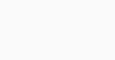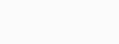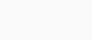Mechanical Basics

# Interview Questions

It is very important to have basic knowledge for cracking the interviews; here I am adding the all subjects interview questions that definitely helps you to score in interviews. Additional to these, please refer the Basics Page for more questions where you can find number of concepts.

Please press and hold the CTRL + F button to open the search bar. In the search bar enter your required Question or Subject name or main keyword of your question to highlight. This  page will be updated frequently with new questions.

For Example: If you want to search for “what is inertia force?” then enter the keyword as Inertia force in search bar.

Subject: MF&HM

Inertia Force is the product of the mass and acceleration of a fluid.

Subject: MF&HM

Viscous Force is the product of shear stress due to viscosity and the cross-sectional area.

Subject: MF&HM

Gravity Force is the product of mass and acceleration due to gravity of the liquid flow.

Subject: MF&HM

Pressure force is the product of intensity of pressure and area of a flowing liquid.

Subject: MF&HM

Surface Tension Force is the product of surface tension per unit length and the length of the surface of flowing liquid.

Subject: MF&HM

Elastic Force is the product of elastic stress and area of flowing liquid.

Crystallization is a process of changing the phase or property of a material. The first one is nucleation, which offers the seems of a crystalline section from both a supercooled liquid or a supersaturated solvent. The 2d step is known as crystal growth, that’s the growth in the length of debris and effects in a crystal state. An important function of this step is that loose particles from layers on the crystal`s floor and resort themselves into open inconsistencies like pores, cracks, etc.

The sum of the atomic masses of all atoms in a molecule; primarily based totally on a scale wherein the atomic loads of hydrogen, carbon, nitrogen, and oxygen are 1, 12, 14, and 16, respectively. Let us calculate the molecular weight of water – Water has 2 hydrogen atoms and one oxygen atom. Therefore, the molecular weight of water is 18 (i.e., 2 + 16). Molecular Weight is also referred to as molecular mass.

Subject: Engineering Drawing

Drawing: A drawing is a rough sketch that uses the information for a particular object.

Drafting: Drafting is a type of drawing that consists of exact scaling, measurements. It does maintain standards like ISO, ANSI, ASME, etc. We can say it is a type of Engineer’s language.

Design: Design is a model of an object or a component that even provide detailed analysis too. It is a final product of estimation. Designing a product include the drafting of components, and analyzing the stresses, forces, etc in all the directions with accurate dimensions.

Subject: Strength of Materials Subject

Sagging and Hogging are the two important concepts in the Strength of Materials; we can find this topic in Bending of Beams.

What is Sagging?• It is the shape of the beam that nulls when we load it from its upside and the beam will bend downward in a concave shape on top and convex shape in bottom side of beam. Such Concave shape in top side is called as the Sagging
• It is also called as the Positive Bending Moment.

What is Hogging?• Hogging is quite opposite to the sagging; it means when a beam is loaded or pressed from the down side then the beam will bend in an opposite direction to the Sagging (upward direction).
• It is the shape of the beam that shows concave shape in Bottom side and convex shape in upside such bending is called as the Hogging in Beam. It is also called as the Negative Bending Moment.

Subject: Strength of Materials Subject

When three mutually perpendicular stresses of equal intensity or load is applied on a body then direct stress will be developed and according to it the strain will be takes place in all the three sides.

So the bulk modulus can be defined as the the ratio of direct stress to the volumetric strain. It is denoted by the letter “K”.Here

sigma V= Change in volume

V=Actual Volume

E=Young’s Modulus

1/m=Poissons ratio

Subject: Strength of Materials Subject

It is defined as the ratio of Lateral Strain to the Linear Strain.

In the problems, Poissons ratio will be given as “x” then we have take as 1/m = 1/x.

Subject: Strength of Materials Subject

Ferrous Metals: Ferrous metals are the material that contain large amount of iron as one of their compositions.

Example: Cast Iron, Steel, etc.

Non-Ferrous Metals: The non-ferrous metals are quite opposite to the ferrous material whereas; it does not contain iron as its composition.

Example: Copper, Zinc, Aluminum, etc.

AutoCAD is a software application for the Computer Aided Drafting.

So here are 12 Important predefined keys called Function Keys uses in the Drafting, they are

• F1: This is the first function where we can see in the top of our keyboard. This is used to open the help window in the AutoCAD.
• F2: This is the key where the AutoCAD will show all the commands which we used previous to draw a object.
• F3: This key is used to On/Off the Osnap feature. To adjust this feature we have to follow the below procedure. Right Click (enter) Settings (enter) EndPoint, MidPoint, Center adjustment.
• F4: This key is use in the 3D drafting where we can On/Off the Osnap feature only in the 3D drafting.
• F5: This key is one of the important feature useful in the isometric drafting.

This function key is used to change the planes to Right, Left, Up and Down sides only in the isometric drafting. To change the window from 2D to Isometric, follow the following procedure. Drafting Settings (DS) (enter) Select the option Isometric from Rectangular.

• F6: This function key is used to On/Off the Grid views.
• F7: This key is a Dynamic User Coordinate Systems (DUCS)used in the 3D.
• F8: This is an another important key, which is used to On/Off the Ortho type where with the switching on this key we will get Straight Lines in the drafting.
• F9: This key is used to On/Off the Snap Grids
• F10: This function key is used to the Track the Polar Objects in the drafing.
• F11: This is key of O-Tracking or Object Tracking. It is used to track the snap point on the objects like line, Rectangle, etc.
• F12: This is a Dynamic Input key where we can give the commands at the place of Cursor instead of Command Bar and also we can see the dimensions at the time of drawing.

Subject: Theory of Machines

The driven gear is the device or a component that depends on the driver, whereas it is not a self-energy generating device.

Subject: Theory of Machines

The driver gear is the source that runs the whole machine with the help of Electricity or any other Fuel; it may be a motor or an engine.

Subject: Theory of Machines

The Gear and Pinion are the two parts that help in transferring the power or energy in a perpendicular direction. Usually, both parts are called Gears but, when they combine to do some work, then the larger part is called the Gear, and the smaller part is called the Pinion

Subject: Strength of Materials

It is a property of the material that the material should be strong, rigid and should not bend with the external loads applied.

Subject: Strength of Materials

It is a property of the material that it should resist the forces and the pressures when applied on it. We do even have an entire subject on Strength of Materials.

Subject: Strength of Materials

It is the property of the material that resist the deformation and remain hard when the forces are applied to it. Materials that possess hardness are like Ceramics, Concrete, etc.

Subject: Strength of Materials

It is defined as the material that is tough and able to absorb the energy and at the same time it should resist the fractures also.

Subject: Strength of Materials

It is the property of the material that can be drawn into the thin sheet and wires. Steel, Aluminum, Copper are some of the ductile materials.

Subject: Strength of Materials

It was just opposite to Toughness where the material will break without any deformation. Cast iron, Glass, Concrete are some of the Brittle materials.

Subject: Strength of Materials

It is the property of the material that can be deformed by compression, expansion and able to regain its original shape. Rubber is one of the examples of the Elasticity.

Subject: Strength of Materials

It is the type of material property which the material gets deform while application of forces and unable to regain its normal shape by its own. Plastic is the best example of the plasticity.

Subject: Strength of Materials

It is the property of the material which it will get weak and fails when repeated loads are applied on it.

Subject: Strength of Materials

It is the property when the material is subjected to heat for much time then it will get deformed.

Subject: Strength of Materials

It is the property of the material that we can mold or can transform into the required shape by applying loads on it.

Subject: Strength of Materials

It is the property of a material where we can twist and bend them without any breakage.

Subject: Heat Transfer

There are three different Modes of Heat transfers; they are Conduction, Convection and Radiation. Each Mode will follow its law so, such laws are called as the governing Laws of rate of Heat Transfer. The laws are called as follow.

1. The law followed by Conduction is called as the Fourier law or Law of Conduction.
2. The law followed by Convection is called as the Newton Law of Cooling or Law of Convection.
3. The law followed by Radiation is called as the Stefan-Boltzmann Law or Law of radiation.

Subject: Heat Transfer

Fourier Law is stated as the rate of heat transfer along given direction is directly proportional to the Temperature gradient and to the area perpendicular to the direction.Qx = -K.A dt/dx

Qx = heat transfer rate

K = Thermal conductivity constant

dt/dx = tempearture gradient  with respect to length

The units of thermal conductivity is watt / meter kelvin

Subject: Heat Transfer

Newton Law of Cooling states that the Rate of Heat transfer is directly proportional to the Temperature difference and the area of the heat transfer.

Q = h. A (Tw – T~)

h = Heat transfer coefficient of convenction.

Tw = Temperature of the Body.

T~ = Ambient temperature or Surrounding air temperature.

The unit of heat transfer coefficient (h) is W/ m^2 K (watt per meter square kelvin).

Subject: Heat Transfer

Stefan-Boltzmann Law states that the electromagnetic wave of block body (Eb) per unit are is directly proportional to the fourth power of the Temperature.The units of Steffan Boltzmann constant is w / m^2  K^4(watt per meter square and fourth power of kelvin)Computer-Aided Design shortly called CAD. CAD is the designing softwares that can able to design the Two dimensional or Three dimensional objects. A few CAD softwares are AutoCAD, Catia, Solidworks, Ansys, etc. CAD will be used only for designing the objects.

Computer Aided Manufacturing shortly called CAM. CAM is the output or manufacturing process of designed component. CAM objects are always three-dimensional. The designed object will consist of coding, such coding is the input for the manufacturing machine. Computer Numerical Machine is one of the examples of CAM machine.

Subject: MF&HM

Answer: 2. Manometric Head – The head developed by a Centrifugal Pump is Proportional to the Diameter & Speed of Impeller.

The Torque is one of the measuring dimension. It is Force that tends to the rotation of any body.

The torque is measured in terms of newtons and meters. The Units of Torque are N-m (call as Newton Meter)

The Acceleration is the dimension or term that is used to measure the speed of a body. It is also defined as the Rate of Change of Speed.

The acceleration is measured in meters and seconds. The Units of Acceleration is m/s2 (call as meter per second square)

It is measured in terms of meters and seconds. Its units are m/s2 (call as meter per second square).

The Friction is defined as the resistance that offers any body or surface while moving with particular speed. The Units of Friction is Newton.

The Force is the action that applies on any body with some energy. The SI Units of Force is Newton.

The Melting Point is the certain temperature number where the metal or any other type of material or metal will start melting when it is continuously heated.